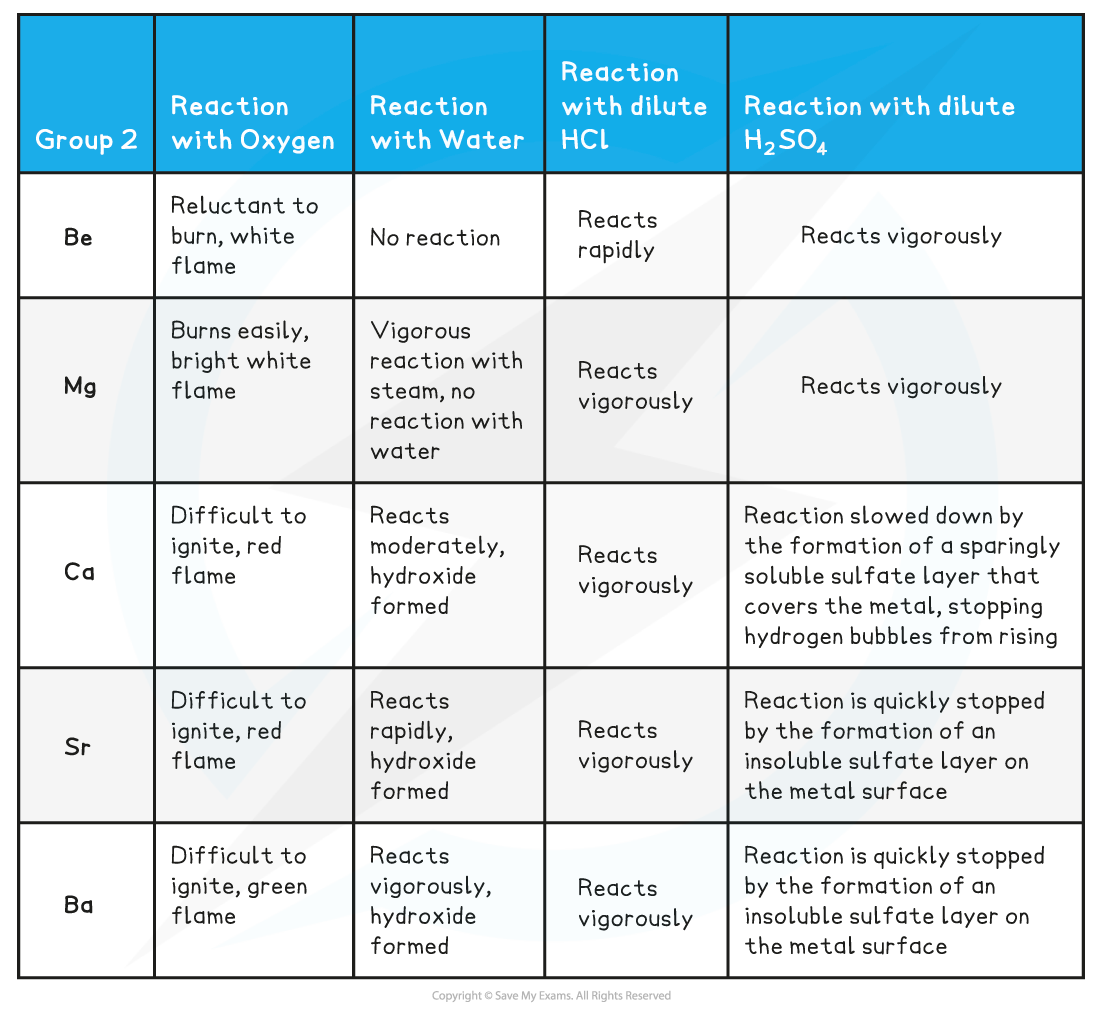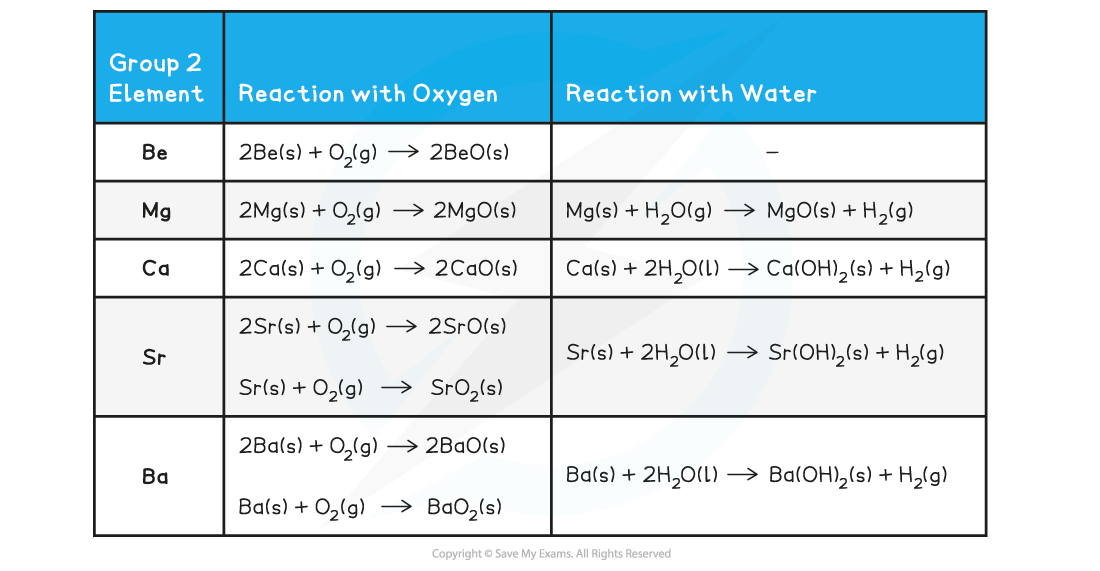# AQA A Level Chemistry复习笔记4.2.1 Reactions of Group 2 Elements

### Reactions of Group 2 Elements

• Although there is no required practical specifically based around the reactions of Group 2 elements, these reactions are important and students tend to not do very well on these questions in the exam
• Practical style questions can be asked on this topic - you should learn this in detail

Reactions of Group 2 Elements• The reaction of all Group 2 metals with oxygen follows the following general equation:

2M (s) + O2 (g) → 2MO (s)

Where M is any metal in Group 2

• Sr and Ba also form MO2
• The reaction of all metals with water follows the following general equation:

M (s) + 2H2O (l) → M(OH)2 (s) + H2 (g)

Except for, Be which does not react with water

• The reaction of all metals with dilute HCl follows the following general equation:

M (s) + 2HCl (aq) → MCl2 (aq) + H2 (g)

• The reaction of all metals with dilute H2SO4 follows the following general equation:

M (s) + H2SO4 (aq) → MSO4 (aq) + H2 (g)

• SrSO4 and BaSO4 are insoluble

Group 2 Reactions with Oxygen & Water Chemical EquationsGroup 2 Reactions with Dilute Hydrochloric Acid & Dilute Sulfuric Acid Chemical Equations